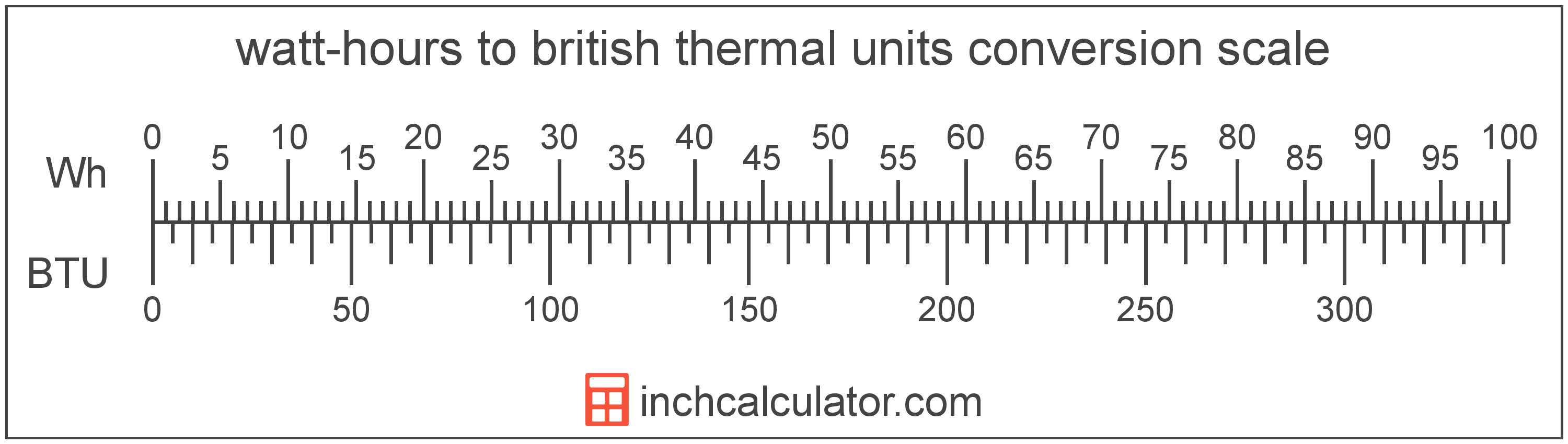# British Thermal Units to Watt-Hours Converter

Enter the energy in british thermal units below to get the value converted to watt-hours.

Results in Watt-Hours:1 BTU = 0.293071 Wh

Do you want to convert watt-hours to british thermal units?

## How to Convert British Thermal Units to Watt-Hours

To convert a measurement in british thermal units to a measurement in watt-hours, multiply the energy by the following conversion ratio: 0.293071 watt-hours/british thermal unit.

Since one british thermal unit is equal to 0.293071 watt-hours, you can use this simple formula to convert:

watt-hours = british thermal units × 0.293071

The energy in watt-hours is equal to the energy in british thermal units multiplied by 0.293071.

For example, here's how to convert 5 british thermal units to watt-hours using the formula above.
watt-hours = (5 BTU × 0.293071) = 1.465355 Wh### How Many Watt-Hours Are in a British Thermal Unit?

There are 0.293071 watt-hours in a british thermal unit, which is why we use this value in the formula above.

1 BTU = 0.293071 Wh

## What is a British Thermal Unit?

British thermal units are a measure of heat energy. Specifically, one british thermal unit is equal to the amount of heat energy required to increase the temperature of one pound of water one degree Fahrenheit. They are often used as a way to rate the energy heat producing appliances such furnaces, and to price heating fuels such as natural gas.

The british thermal unit is a US customary unit of energy. British thermal units can be abbreviated as BTU; for example, 1 british thermal unit can be written as 1 BTU.

## What is a Watt-Hour?

The watt-hour is a measure of electrical energy equal to one watt of power over a one hour period.

Watt-hours are usually abbreviated as Wh, although the formally adopted expression is W·h. The abbreviation W h is also sometimes used. For example, 1 watt-hour can be written as 1 Wh, 1 W·h, or 1 W h.

In formal expressions, the centered dot (·) or space is used to separate units used to indicate multiplication in an expression and to avoid conflicting prefixes being misinterpreted as a unit symbol.

## British Thermal Unit to Watt-Hour Conversion Table

Table showing various british thermal unit measurements converted to watt-hours.
British Thermal Units Watt-hours
1 BTU 0.293071 Wh
2 BTU 0.586142 Wh
3 BTU 0.879213 Wh
4 BTU 1.1723 Wh
5 BTU 1.4654 Wh
6 BTU 1.7584 Wh
7 BTU 2.0515 Wh
8 BTU 2.3446 Wh
9 BTU 2.6376 Wh
10 BTU 2.9307 Wh
11 BTU 3.2238 Wh
12 BTU 3.5169 Wh
13 BTU 3.8099 Wh
14 BTU 4.103 Wh
15 BTU 4.3961 Wh
16 BTU 4.6891 Wh
17 BTU 4.9822 Wh
18 BTU 5.2753 Wh
19 BTU 5.5684 Wh
20 BTU 5.8614 Wh
21 BTU 6.1545 Wh
22 BTU 6.4476 Wh
23 BTU 6.7406 Wh
24 BTU 7.0337 Wh
25 BTU 7.3268 Wh
26 BTU 7.6198 Wh
27 BTU 7.9129 Wh
28 BTU 8.206 Wh
29 BTU 8.4991 Wh
30 BTU 8.7921 Wh
31 BTU 9.0852 Wh
32 BTU 9.3783 Wh
33 BTU 9.6713 Wh
34 BTU 9.9644 Wh
35 BTU 10.26 Wh
36 BTU 10.55 Wh
37 BTU 10.84 Wh
38 BTU 11.14 Wh
39 BTU 11.43 Wh
40 BTU 11.72 Wh

## References

1. Bureau International des Poids et Mesures, The International System of Units (SI), 9th edition, 2019, https://www.bipm.org/documents/20126/41483022/SI-Brochure-9-EN.pdf